# Image Classifier: Error Rate Increases after Updating Learning Rate

For 4 epochs, I had a low error rate of 5.2%. However, after updating the learning rate while training, the error rate has gone up to 18.4%. Why does this happen?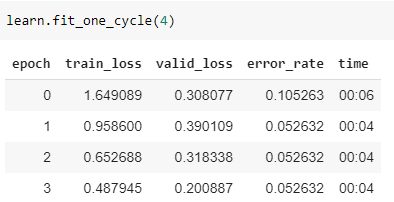\leftarrow Before updating the learning rate.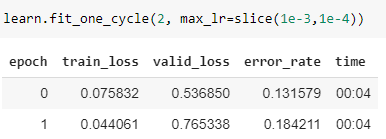\leftarrow After updating the learning rate.

At first, the learning rate plot did not appear when I ran-

learn.recorder.plot()


I just received an empty plot.
Then, when I ran, as advised-

learn.lr_find(start_lr=1e-5, end_lr=1e-1)
learn.recorder.plot()


Then I got this-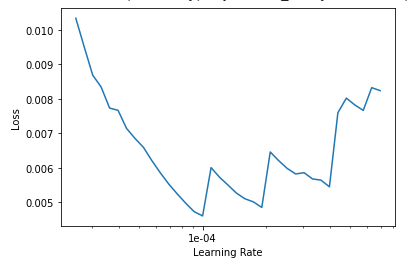So, when I ran the code with the learning rate specified, I got my errors increased.

Why does this happen? How to solve this problem?

first, just run learn.lr_find() without params
second, your slice is (1e-3, 1e-4). it should be (1e-4, 1e-3). try that.

1 Like

Running learn.lr_find() gave me an empty plot.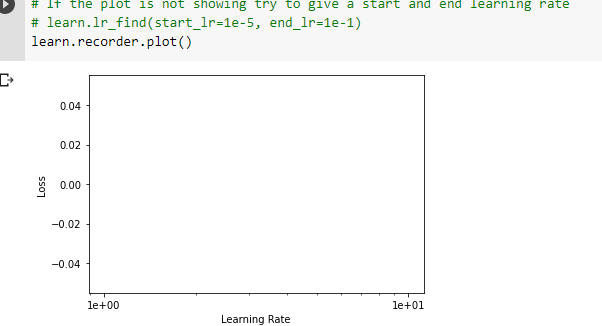When I specified as advised, it worked.

And the silly mistake of putting 1e-3 was causing the problem. Switched it, and that solved the problem.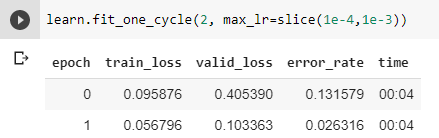1 Like